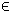## 28MCP Problem

TOMLAB requires that general nonlinear complementarity problems are defined in Matlab m-files. The function to be optimized does not need to be supplied (pure equilibrium problem). It is recommended that the user supply as many analytical functions as possible.

The constrained nonlinear complementarity problem is defined as:

 min x
f(x)

s/t
 xL ≤ x ≤ xU bL ≤ A x ≤ bU cL ≤ c(x) ≤ cU
(32)
where x, xL, xURn, f(x)R, ARm1 × n, bL,bURm1 and cL,c(x),cURm2.

The complementarity conditions can be any combination of decision variables and linear/nonlinear constraints:
• x(i) ⊥ x(j)
• x(i) ⊥ A(j,:) x
• x(i) ⊥ c(j)
• A(i,:) xA(j,:) x
• A(i,:) xc(j)
• c(i) ⊥ c(j)
The following files define the problem in TOMLAB.

File: tomlab/quickguide/mcpQG_f.m, mcpQG_g.m, mcpQG_H.m, mcpQG_c.m, mcpQG_dc.m, mcpQG_d2c.m
```  f:   Function value
H:   Hessian matrix
c:   Nonlinear constraint vector
d2c: The second part of the Hessian to the Lagrangian function for the nonlinear constraints.
```
The following file illustrates how to solve a MCP problem in TOMLAB. Also view the m-files specified above for more information.

File: tomlab/quickguide/mcpQG.m

Open the file for viewing, and execute mcpQG in Matlab.
```% mcpQG.m
%
% Demonstrates how to setup and solve a nonlinear problem with equilibrium
% (complementary) constraints.
%
% TOMLAB /KNITRO is capable of solving general complementarity problems
%
% The problem is the following:
%
% minimize  f = (x(1)-5)^2 + (2*x(2)+1)^2
%
% subject to
%
%    2*(x(2)-1) - 1.5*x(1) + x(3) - 0.5*x(4) + x(5) == 0   % c(1)
%     3*x(1) - x(2) - 3     >= 0                           % c(2)
%      -x(1) + 0.5*x(2) + 4 >= 0                           % c(3)
%      -x(1) -     x(2) + 7 >= 0                           % c(4)
%
%    x(1:5) >= 0
%
% The following complementarity demands are also imposed:
%
%   0 <= c(2) _|_ x(3) >= 0
%   0 <= c(3) _|_ x(4) >= 0
%   0 <= c(4) _|_ x(5) >= 0
%
% All four constraints are in fact linear, but are modelled as nonlinear
% constraints with the constants moved to the LHS.
%
% The same problem is solved as a explicitly quadratic complementarity
% problem in qcpQG.m

% There are no (explicitly) linear constraints.
A   = [];
b_L = [];
b_U = [];

% Provide a pattern for the nonlinear constraints
ConsPattern = [
1 1 1 1 1
1 1 1 0 0
1 1 0 0 0
1 1 0 0 0
];

HessPattern = [];

% First constraint is equality == 0, the remaining are >= 0
c_L = [0,0,0,0]';
c_U = [0,inf,inf,inf]';

x_L = zeros(5,1);
x_U = inf*ones(5,1);
x_0 = x_L;

Name = 'JF Bard 1998 MPEC';

% Functions for calculating the nonlinear function and derivative values.
f   = 'mcpQG_f';
g   = 'mcpQG_g';
H   = 'mcpQG_H';
c   = 'mcpQG_c';
dc  = 'mcpQG_dc';
d2c = 'mcpQG_d2c';

% Each row of mpec is one pair. The first says x(3) _|_ c(2) should be
% complementary. Exactly two nonzeros per row is allowed.

mpec = [ ...
3,0, 0,0, 2,0; ...
4,0, 0,0, 3,0; ...
5,0, 0,0, 4,0; ...
];

Prob = mcpAssign(f, g, H, HessPattern, x_L, x_U, Name, x_0, mpec, ...
[], ...
A, b_L, b_U, c, dc, d2c, ConsPattern, c_L, c_U);

Prob.PriLevOpt = 2;

% KNITRO options. Algorithm 3 works good on this problem.
opts     = [];
opts.ALG = 3;
Prob.KNITRO.options = opts;

% Now solve this problem with KNITRO:
Result = tomRun('knitro',Prob,2);

% The original problem is available as Prob.orgProb.

N0 = Prob.orgProb.N;

x = Result.x_k;
s = x(N0+1:end)     % The slacks added by BuildMPEC
x = x(1:N0)         % The "original" variables

% Constraint values for the modified problem. If not infeasible, these
% should all be zero because BuildMPEC has changed the constraints in the
% mpec pairs to include slack variables.

c = Result.c_k

% The permutation matrix for the slacks in the nonlinear constraints is
% available:

MP = full(Prob.MPEC.MP);

% The values of the constraints + their respective slacks:

C = c + MP*s

x(3:5), C(2:4)

% This should give something like:
%
% ans =
%
%     3.5000
%          0
%          0
%
%
% ans =
%
%      0
%      3
%      6
%
% I.e, we have the complementarity conditions satisified.```